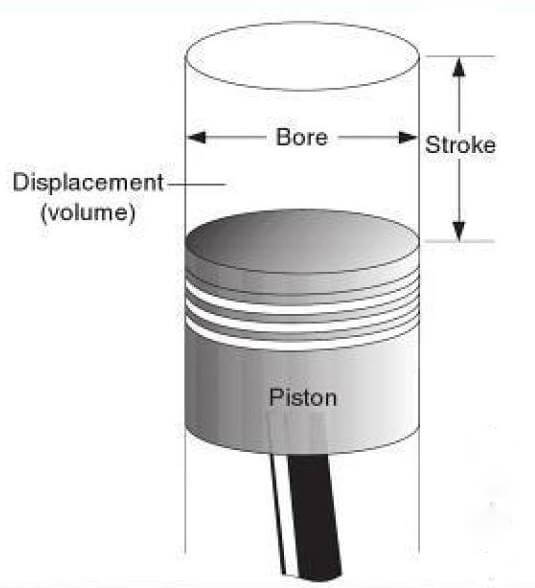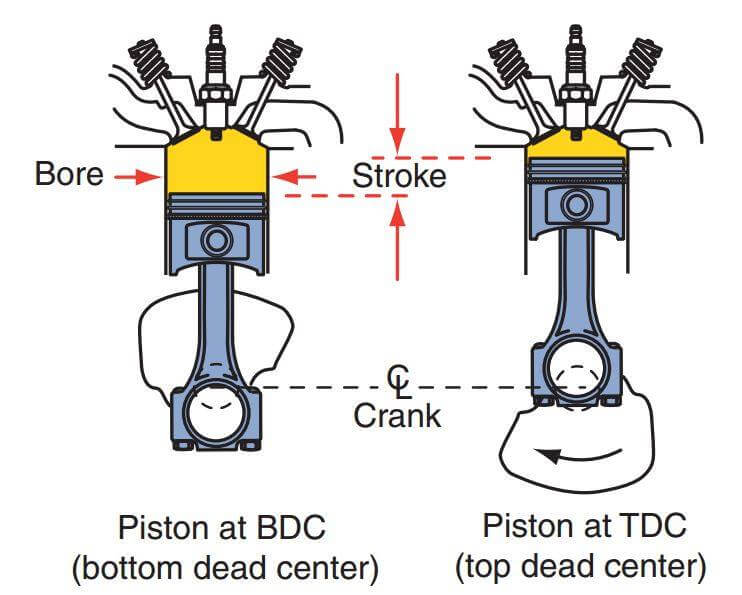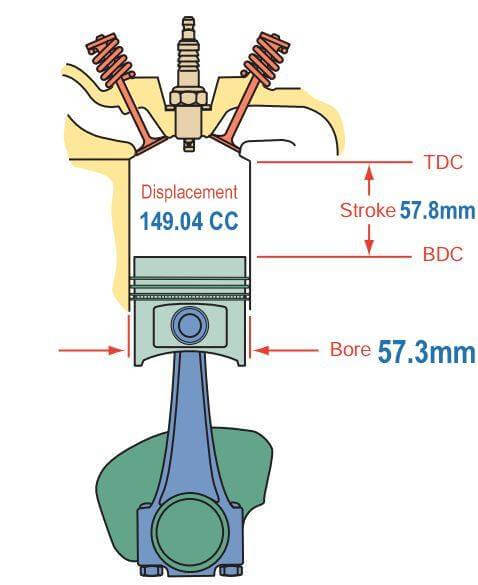## BASIC MOTORCYCLE ENGINES THEORY AND MATH (Part-01)

Published On 07-Nov-2013 06:13am , By Shuvo

Engine Displacement VolumeIn this illustration, you can see the bore and stroke of  motorcycle engines. This information is used to determine the engine displacement Volume: Volume is also a measurement of size and is related to mass and weight. Volume is the amount of space occupied by an object in three dimensions: length, width, and height. For example, a pound of gold and a pound of feathers both have the same weight, but the pound of feathers occupies a much larger volume. In the English system, volume is measured in cubic inches, cubic feet, cubic yards, or gallons. The measurement for volume in the metric system is cubic centimeters or liters the volume of a container is calculated by taking an object’s measured length, width, and height and multiplying them. For example, if a box has a length of 2 inches, a width of 3 inches, and a height of 4 inches (2 ×3 ×4 =24), its volume equals 24 cubic inches.Different shapes have different formulas for calculating volume but all consider the object’s three dimensions. The volume of an engine’s cylinders is expressed as displacement. This size does not reflect the external (length, width, and height) of the engine. Cylinder displacement is the maximum volume of a cylinder.  A piston’s travel from its lowest point (BDC) to its highest point (TDC) within the cylinder is called the stroke of the piston. A cylinder’s bore is the diameter of the cylinder. Displacement is usually measured in cubic inches, cubic centimeters, or liters. The total displacement of an engine (including all cylinders) is a rough indicator of its power output. Total displacement is the sum of displacements for all cylinders in an engine. Engine cubic inch displacement (CID) may be calculated as follows: CID=π× R²× L × NWhere π =3.1416 R =the radius of the cylinder or (the diameter, the bore, ÷2) L =length of stroke N=number of cylinders in the engine Example: Calculate the CID of a single-cylinder motorcycle engine with a 57.3mm. bore and 57.8mm. stroke. [Calculate the metric displacement of a single-cylinder engine with a 57.3mm. bore and 57.8 mm. stroke. Before you use the formula to find the displacement in cubic centimeters, convert the millimeter measurements to centimeters: Bore 57.3mm=5.73cm, Stroke 57.8mm =5.78cm (1mm=10cm)] CID =3.1416 ×2.865²×5.78×1 CID= 149.04 cubic centimeters (cc) Most of today’s motorcycle engines listed by their metric displacement. Cubic centimeters and liters are determined by using metric measurements in the displacement formula. By-A.K.M.ABIDUR RAHMAN

Best BikesPrice: 169800Price: 255000Price: 200900

Latest BikesPrice: 0Price: 0Price: 0

Upcoming BikesPrice: 0Price: 0Price: 0